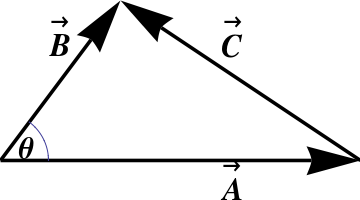## Section1.9The Law of CosinesFigure 1.9.1. The Law of Cosines is just the definition of the dot product.

The definition of the dot product can be used to prove several familiar formulas. For example, consider Figure 1.9.1, in which $$\CC=\BB-\AA\text{.}$$ Then

\begin{align*} \CC\cdot\CC \amp = (\BB -\AA) \cdot (\BB -\AA) \nonumber\\ \amp = \AA\cdot\AA + \BB\cdot\BB - 2 \,\AA\cdot\BB \end{align*}

or equivalently

\begin{equation} \,|\CC|^2 = |\AA|^2 + |\BB|^2 - 2|\AA||\BB|\cos\theta\tag{1.9.1} \end{equation}

which is just the Law of Cosines.